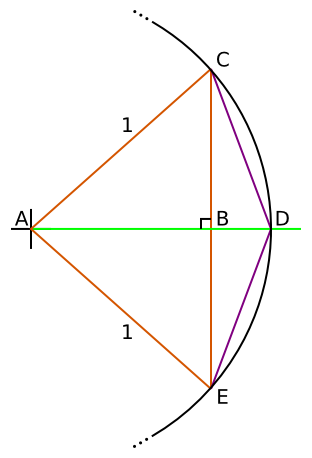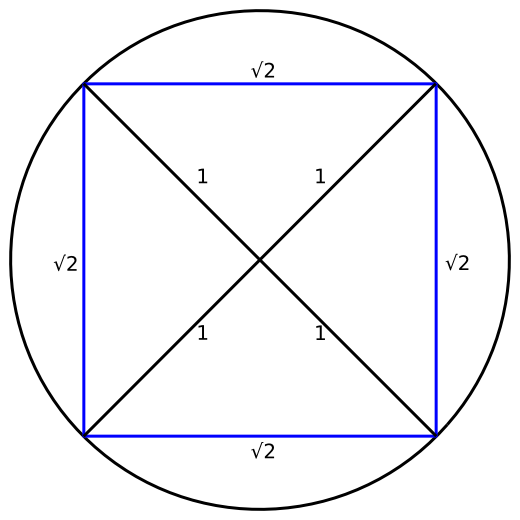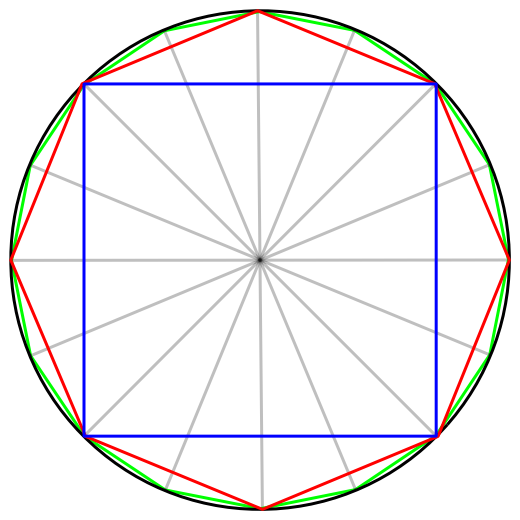Pi - Archimedes

2011-07-18

It is clear from Part 1 that in order to calculate π we are going to need a better method than evaluating Gregory's Series.

Here is one which was originally discovered by Archimedes in 200BC. Most of the proofs for series for calculating π require calculus or other advanced math, but since Archimedes didn't know any of those you can prove it without! In fact I thought I had discovered it for the first time when I was 13 or so, but alas I was 2000 years too late!

Archimedes knew that the ratio of the circumference of a circle to its diameter was π. He had the idea that he could draw a regular polygon inscribed within the circle to approximate π, and the more sides he drew on the polygon, the better approximation to π he would get. In modern maths speak, we would say as the number of sides of the polygon tends to infinity, the circumference tends to 2π.

Instead of drawing the polygon though, Archimedes calculated the length using a geometric argument, like this.Draw a circle of radius 1 unit centered at A. Inscribe an N sided polygon within it. Our estimate for π is half the circumference of the polygon (circumference of a circle is 2πr, r = 1, giving 2π). As the sides of the polygon get smaller and smaller the circumference gets closer and closer to 2π.

The diagram shows one segment of the polygon ACE. The side of the polygon CE has length d n. Assuming we know d for an N sided polygon, If we can find an expression for the length CD = d 2n, the edge length of a polygon with 2N sides, in terms of d n only, then we can improve our estimate for π. Let's try to do that.

We bisect the triangle CAE to make CAD and DAE the two new equal segments of the 2N sided polygon.

Using Pythagoras's theorem on the right angled triangle ABC, we can see:Given thatSubstituting givesUsing Pythagoras's theorem on the right angled triangle CBDd 2n is the length of one side of the polygon with 2N sides.

This means that if we know the length of the sides of a polygon with N sides, then we can calculate the length of the sides of a polygon with 2N sides.

What does this mean? Lets start with a square. Inscribing a square in a circle looks like this, with the side length √2. This gives an estimate for π as 2√2, which is poor (at 2.828) but it is only the start of the process.We can the calculate the side length of an octagon, from the side length of a square, and the side length of a 16-gon from an octagon etc.It's almost time to break out python, but before we do so, we'll just note that to calculate d 2n from d n, the first thing you do is calculate d² and at the very end you take the square root. This kind of suggests that it would be better to keep track of d² rather than d, which we do in the program below (pi_archimedes.py):

import math

def pi_archimedes(n):
"""
Calculate n iterations of Archimedes PI recurrence relation
"""
polygon_edge_length_squared = 2.0
polygon_sides = 4
for i in range(n):
polygon_edge_length_squared = 2 - 2 * math.sqrt(1 - polygon_edge_length_squared / 4)
polygon_sides *= 2
return polygon_sides * math.sqrt(polygon_edge_length_squared) / 2

def main():
"""
Try the series
"""
for n in range(16):
result = pi_archimedes(n)
error = result - math.pi
print("%8d iterations %.10f error %.10f" % (n, result, error))

if __name__ == "__main__":
main()

If you run this, then it produces:

Iterations Sides Result Error
0 4 2.8284271247 -0.3131655288
1 8 3.0614674589 -0.0801251947
2 16 3.1214451523 -0.0201475013
3 32 3.1365484905 -0.0050441630
4 64 3.1403311570 -0.0012614966
5 128 3.1412772509 -0.0003154027
6 256 3.1415138011 -0.0000788524
7 512 3.1415729404 -0.0000197132
8 1024 3.1415877253 -0.0000049283
9 2048 3.1415914215 -0.0000012321
10 4096 3.1415923456 -0.0000003080
11 8192 3.1415925765 -0.0000000770
12 16384 3.1415926335 -0.0000000201
13 32768 3.1415926548 0.0000000012
14 65536 3.1415926453 -0.0000000083
15 131072 3.1415926074 -0.0000000462

Hooray! We calculated π to 8 decimal places in only 13 iterations. Iteration 0 for the square shows up the expected 2.828 estimate for π. You can see after iteration 13 that the estimate of π starts getting worse. That is because we only calculated all our calculations to the limit of precision of python's floating point numbers (about 17 digits), and all those errors start adding up.

We can easily calculate more digits of π using Pythons excellent decimal module. This allows you to do arbitrary precision arithmetic on numbers. It isn't particularly quick, but it is built in and easy to use.

Let's calculate π to 100 decimal places now. That sounds like a significant milestone! (pi_archimedes_decimal.py):

from decimal import Decimal, getcontext

def pi_archimedes(n):
"""
Calculate n iterations of Archimedes PI recurrence relation
"""
polygon_edge_length_squared = Decimal(2)
polygon_sides = 2
for i in range(n):
polygon_edge_length_squared = 2 - 2 * (1 - polygon_edge_length_squared / 4).sqrt()
polygon_sides *= 2
return polygon_sides * polygon_edge_length_squared.sqrt()

def main():
"""
Try the series
"""
places = 100
old_result = None
for n in range(10*places):
# Do calculations with double precision
getcontext().prec = 2*places
result = pi_archimedes(n)
# Print the result with single precision
getcontext().prec = places
result = +result           # do the rounding on result
print("%3d: %s" % (n, result))
if result == old_result:
break
old_result = result

You'll see if you look at the pi_archimedes function that not a lot has changed. Instead of using the math.sqrt function we use the sqrt method of the Decimal object. The edge gets initialised to Decimal(2) rather than 2.0 but otherwise the methods are the same. The main method has changed a bit. You'll see that we set the precision of the decimal calculations using the getcontext().prec = ... call. This sets the precision for all following calculations. There are other ways to do this which you can see in the decimal module docs. We do the Archimedes calculations with 200 decimal places precision, then print the result out with 100 decimal places precision by changing the precision and using the result = +result trick. When the result stops changing we end, because that must be π!

If you run this, you'll find at iteration 168 it produces 100 accurately rounded decimal places of π. So far so good!

There are two downsides to this function though. One is that the decimal arithmetic is quite slow. On my computer it takes about 6 seconds to calculate 100 digits of π which sounds fast, but if you were to try for 1,000,000 places you would be waiting a very very long time! The other problem is the square root. Square roots are expensive operations, they take lots of multiplications and divisions and we need to do away with that to go faster.

In Part 3 we'll be getting back to the infinite series for arctan and on to much larger numbers of digits of π.

Categories: maths, pymath, python | View Comments